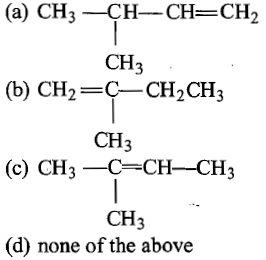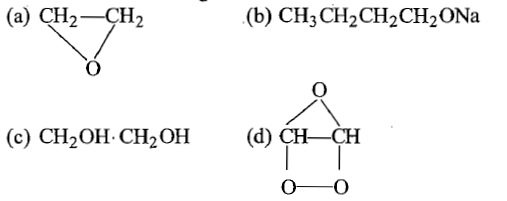Which is an excellent antiseptic?

(a) Phenol                                    (b) Benzyl alcohol

(c) Benzaldehyde                          (d) Acetic acid

Concept Questions :-

Alcohols: Preparation, Physical and Chemical Properties
High Yielding Test Series + Question Bank - NEET 2020

Difficulty Level:

The dehydration of neo-pentanol gives mainly:Concept Questions :-

Alcohols: Preparation, Physical and Chemical Properties
High Yielding Test Series + Question Bank - NEET 2020

Difficulty Level:

Which one of the following on oxidation gives a ketone?

1. Primary alcohol                      2. Secondary alcohol

3. Tertiary alcohol                      4. All of these

Concept Questions :-

Alcohols: Preparation, Physical and Chemical Properties
High Yielding Test Series + Question Bank - NEET 2020

Difficulty Level:

In the following sequence the product (C) is:

CH3CHO$\underset{\mathrm{Pd}}{\overset{{\mathrm{H}}_{2}}{\to }}$ (A) $\stackrel{\mathrm{Na}}{\to }$(B) $\stackrel{{\mathrm{CH}}_{3}\mathrm{I}}{\to }$(C)

1. alcohol                                 2. ether

3. alkene                                  4. none of these

Concept Questions :-

Alcohols: Preparation, Physical and Chemical Properties
High Yielding Test Series + Question Bank - NEET 2020

Difficulty Level:

1. 48% alcohol by mass

2. 10% alcohol by mass

3. 5% alcohol by mass

4. 90% alcohol by mass

Concept Questions :-

Alcohols: Preparation, Physical and Chemical Properties
High Yielding Test Series + Question Bank - NEET 2020

Difficulty Level:

The correct order of solubility of 1$°$,2$°$ and 3$°$ alcohol in water is:

(a) 3$°$>2$°$>1$°$                              (b) 1$°$>2$°$>3$°$

(c) 3$°$>1$°$>2$°$                              (d) none of these

Concept Questions :-

Alcohols: Preparation, Physical and Chemical Properties
High Yielding Test Series + Question Bank - NEET 2020

Difficulty Level:

Which of the following is an alkoxide?Concept Questions :-

Ethers: Preparation, Physical & Chemical Properties, Uses
High Yielding Test Series + Question Bank - NEET 2020

Difficulty Level:

The value of C-O-C angle in ether molecule is :

1. 180$°$

2. 150$°$

3. 90$°$

4. 110$°$

Concept Questions :-

Ethers: Preparation, Physical & Chemical Properties, Uses
High Yielding Test Series + Question Bank - NEET 2020

Difficulty Level:

Ethyl alcohol is also known as:

1. spirit of wine

2. methyl carbinol

3. grain alcohol

4. all of these

Concept Questions :-

Alcohols: Preparation, Physical and Chemical Properties
High Yielding Test Series + Question Bank - NEET 2020

Difficulty Level:

The products formed in the following reaction, C6H5-O-CH3 + HI $\stackrel{\mathrm{Heat}}{\to }$ are:

(a) C6H5OH and CH3I                 (b) C6H5I and CH3OH

(C) C6H5CH3 and HOI                 (d) C6H6 and CH3OI

Concept Questions :-

Phenols: Preparation, Properties, Acidic Nature of Phenol